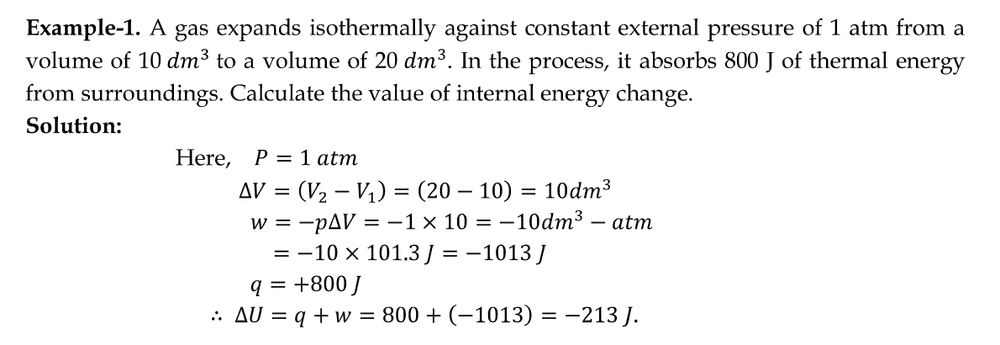# First law of thermodynamics and the work done on a system

Hamiltonian
The first law of thermodynamics states that the change in the internal energy of a system equals the net heat transfer into the system minus the net work done by the system. In equation form, the first law of thermodynamics is ΔU = Q − W.
in the mathematical expression of the first law of thermodynamics, I do not understand the significance of W or the net work done on the system. shouldn't simply be ΔU = Q, if Q is the net heat transfer into the system.

Clearly I am missing something very important hear but all the sources that I have referred to so far just mention the above expression and don't exactly explain W so is this something so obvious that isn't worth explicitly explaining?

Homework Helper
Gold Member
2022 Award
It's much the same idea as the first law of banking-dynamics: the change in your bank balance equals the amount of money put in minus the amount of work your bank account does (i.e. the bills you pay). We'd all like ##\Delta U = Q## for our bank balances, but unfortunately you can't get rid of the ##-W##.

•russ_watters
Hamiltonian
It's much the same idea as the first law of banking-dynamics: the change in your bank balance equals the amount of money put in minus the amount of work your bank account does (i.e. the bills you pay). We'd all like ##\Delta U = Q## for our bank balances, but unfortunately you can't get rid of the ##-W##.
what exactly is the physical significance of ##-W## in the first law thermodynamics?
why does the system have to do this work and on what exactly is it doing it on?

Homework Helper
Gold Member
2022 Award
what exactly is the physical significance of ##-W## in the first law thermodynamics?
why does the system have to do this work and on what exactly is it doing it on?
It doesn't have to do work, but if it does you have to take that into account.

Hamiltonian
It doesn't have to do work, but if it does you have to take that into account.here shouldn't ##\Delta##U = 800J. why is w being added here, also here change in internal energy is -213J, internal energy in itself can never be -ve?

Gold Member
2022 Award
Of course, both "heat" and "work" are forms of energy. The distinction is that the former is the change of energy in the "unordered" (thermal) motion of the particles in the rest frame of the fluid and the latter is the change due to the "ordered" (collective) motion of the fluid as a whole.

Mentor
What is your understand about what work represents? Do you think that a gas is capable of doing work on something else? If so, how?

•vanhees71
Hamiltonian
What is your understand about what work represents? Do you think that a gas is capable of doing work on something else? If so, how?
If a container contains a gas and it is closed on top by a piston(light) then the pressure inside the container will push the piston up(if the pressure inside is higher) and hence do work?

Hamiltonian
Of course, both "heat" and "work" are forms of energy. The distinction is that the former is the change of energy in the "unordered" (thermal) motion of the particles in the rest frame of the fluid and the latter is the change due to the "ordered" (collective) motion of the fluid as a whole.
I don't understand what you mean by unordered motion and ordered motion.

Mentor
If a container contains a gas and it is closed on top by a piston(light) then the pressure inside the container will push the piston up(if the pressure inside is higher) and hence do work?
That is correct. Do you think that the gas doing this amount of work would somehow affect the internal energy of the gas?

•Hamiltonian
Hamiltonian
That is correct. Do you think that the gas doing this amount of work would somehow affect the internal energy of the gas?
yes, it would decrease its internal energy.
In the example mentioned above the 800J of energy absorbed is unrelated to the pressure applied i.e. the energy is supplied is from an arbitrary external source?

Mentor
yes, it would decrease its internal energy.
In the example mentioned above the 800J of energy absorbed is unrelated to the pressure applied i.e. the energy is supplied is from an arbitrary external source?
Yes. If it wasn't added, the internal energy of the gas would decrease even more. It offsets part of the internal energy decrease caused by the gas doing the work.

•Hamiltonian
Gold Member
2022 Award
I don't understand what you mean by unordered motion and ordered motion.
A gas consists of very many atoms/molecules. Start with a gas in thermal equilibrium. As a whole it's at rest but each individual molecule moves around and scattering with the other molecules and the wall of the container. In thermal equilibrium these motions can be described statistically. On the average each gas molecule is at rest but it is moving around colliding with other molecules. The kinetic energy contained in this random motion is the internal energy of the gas.

Now you can change this internal energy on the one hand by just heating the gas up or cooling it down, i.e., you change the kinetic energy of the random motion of the molecules. That's transfer of heat.

On the other hand you can also take your example with the piston where the gas does work by an "ordered motion" of the piston thereby expanding as a whole (collective motion). As you correctly stated above the corresponding energy is taken from the gas. So you can change the state of the gas by both transferring heat as well as doing mechanical work.

•Hamiltonian
•